# bild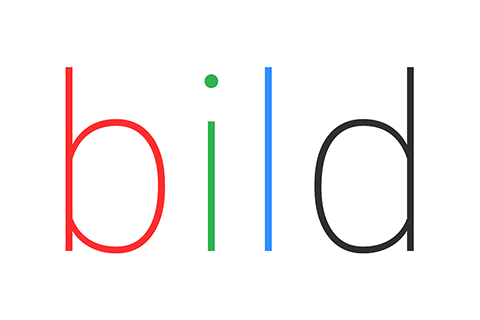A collection of parallel image processing algorithms in pure Go.

The aim of this project is simplicity in use and development over absolute high performance, but most algorithms are designed to be efficient and make use of parallelism when available.

It uses packages from the standard library whenever possible to reduce dependency use and development abstractions.

All operations return image types from the standard library.

## Documentation

The documentation for the various packages is available here.

## CLI usage

``````go get github.com/anthonynsimon/bild
``````

Or get the pre-compiled binaries for your platform on the releases page

``````bild

A collection of parallel image processing algorithms in pure Go

Usage:
bild [command]

Available Commands:
blend       blend two images together
blur        blur an image using the specified method
channel     channel operations on images
effect      apply effects on images
histogram   histogram operations on images
imgio       i/o operations on images
noise       noise generators
segment     segment an image using the specified method

Flags:
-h, --help      help for bild
--version   version for bild

``````

For example, to apply a median effect with a radius of 1.5 on the image `input.png`, writing the result into a new file called `output.png`:

``````bild effect median --radius 1.5 input.png output.png
``````

## Install package

bild requires Go version 1.11 or greater.

```go get github.com/anthonynsimon/bild/...
```

## Basic package usage example:

```package main

import (
"github.com/anthonynsimon/bild/effect"
"github.com/anthonynsimon/bild/imgio"
"github.com/anthonynsimon/bild/transform"
)

func main() {
img, err := imgio.Open("input.jpg")
if err != nil {
fmt.Println(err)
return
}

inverted := effect.Invert(img)
resized := transform.Resize(inverted, 800, 800, transform.Linear)
rotated := transform.Rotate(resized, 45, nil)

if err := imgio.Save("output.png", rotated, imgio.PNGEncoder()); err != nil {
fmt.Println(err)
return
}
}
```

# Output examples

``````import "github.com/anthonynsimon/bild/adjust"
``````

### Brightness

``````result := adjust.Brightness(img, 0.25)
``````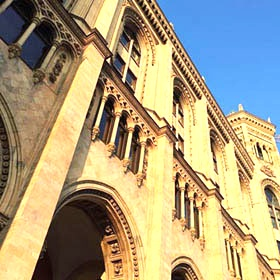### Contrast

``````result := adjust.Contrast(img, -0.5)
``````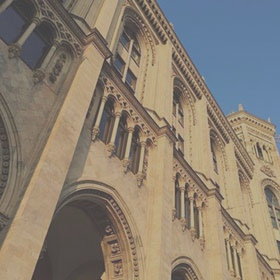### Gamma

``````result := adjust.Gamma(img, 2.2)
``````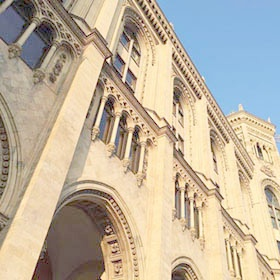### Hue

``````result := adjust.Hue(img, -42)
``````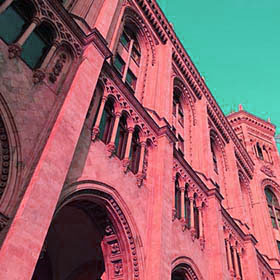### Saturation

``````result := adjust.Saturation(img, 0.5)
``````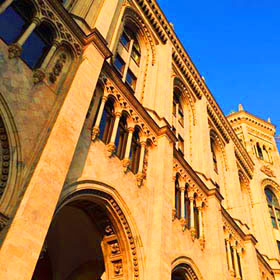## Blend modes

``````import "github.com/anthonynsimon/bild/blend"

result := blend.Multiply(bg, fg)
``````

## Blur

``````import "github.com/anthonynsimon/bild/blur"
``````

### Box Blur

``````result := blur.Box(img, 3.0)
``````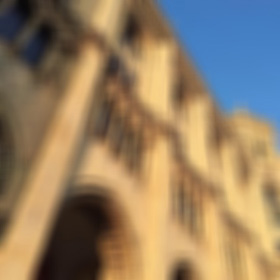### Gaussian Blur

``````result := blur.Gaussian(img, 3.0)
``````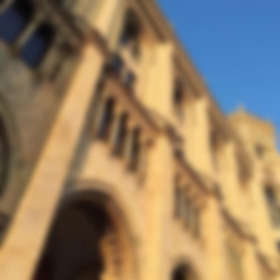## Channel

``````import "github.com/anthonynsimon/bild/channel"
``````

### Extract Channels

``````result := channel.Extract(img, channel.Alpha)
``````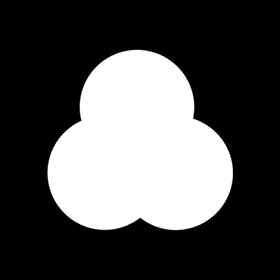### Extract Multiple Channels

``````result := channel.ExtractMultiple(img, channel.Red, channel.Alpha)
``````

## Effect

``````import "github.com/anthonynsimon/bild/effect"
``````

### Dilate

``````result := effect.Dilate(img, 3)
``````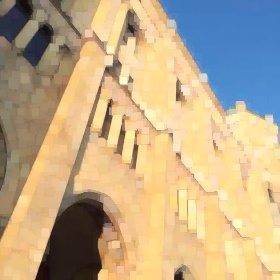### Edge Detection

``````result := effect.EdgeDetection(img, 1.0)
``````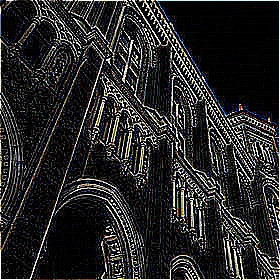### Emboss

``````result := effect.Emboss(img)
``````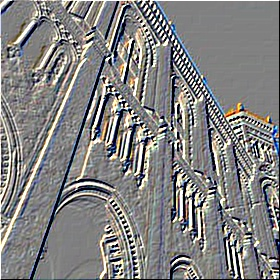### Erode

``````result := effect.Erode(img, 3)
``````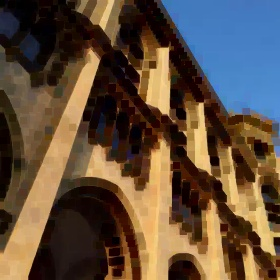### Grayscale

``````result := effect.Grayscale(img)
``````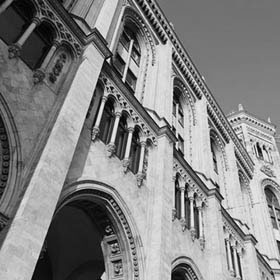### Invert

``````result := effect.Invert(img)
``````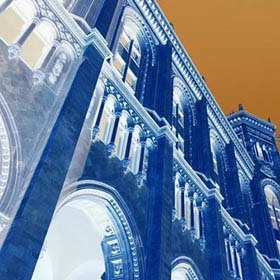### Median

``````result := effect.Median(img, 10.0)
``````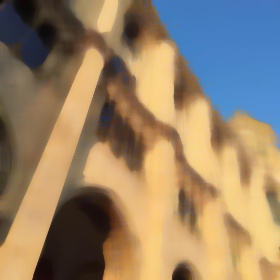### Sepia

``````result := effect.Sepia(img)
``````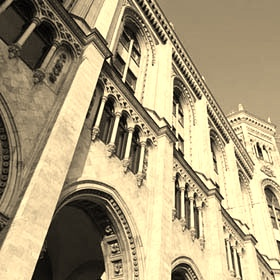### Sharpen

``````result := effect.Sharpen(img)
``````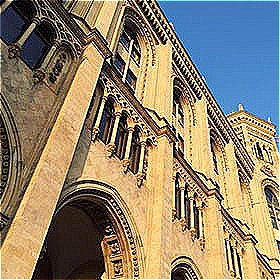### Sobel

``````result := effect.Sobel(img)
``````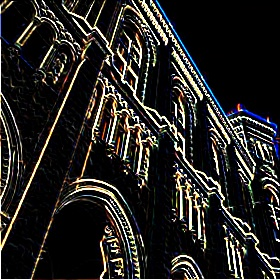``````result := effect.UnsharpMask(img, 0.6, 1.2)
``````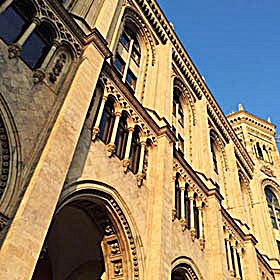## Histogram

``````import "github.com/anthonynsimon/bild/histogram"
``````

### RGBA Histogram

``````hist := histogram.NewRGBAHistogram(img)
result := hist.Image()
``````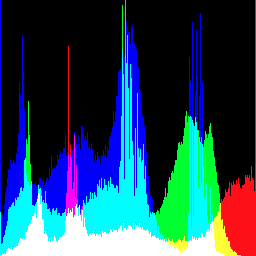## Noise

``````import "github.com/anthonynsimon/bild/noise"
``````

### Uniform colored

``````result := noise.Generate(280, 280, &noise.Options{Monochrome: false, NoiseFn: noise.Uniform})
``````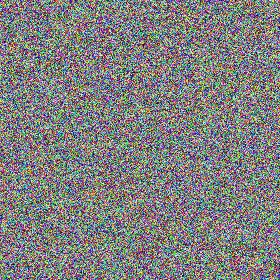### Binary monochrome

``````result := noise.Generate(280, 280, &noise.Options{Monochrome: true, NoiseFn: noise.Binary})
``````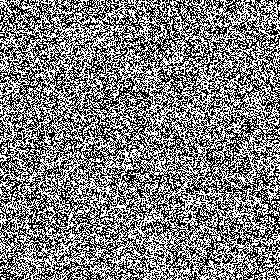### Gaussian monochrome

``````result := noise.Generate(280, 280, &noise.Options{Monochrome: true, NoiseFn: noise.Gaussian})
``````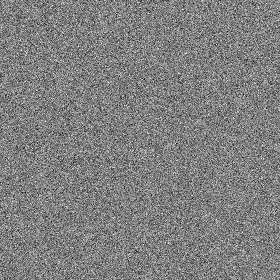### Perlin Noise

``````result := noise.GeneratePerlin(280, 280, 0.25)
``````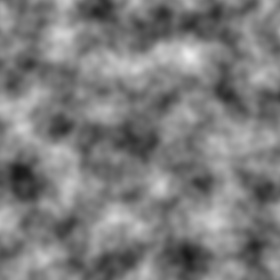## Paint

``````import "github.com/anthonynsimon/bild/paint"
``````

### Flood Fill

``````// Fuzz is the percentage of maximum color distance that is tolerated
result := paint.FloodFill(img, image.Point{240, 0}, color.RGBA{255, 0, 0, 255}, 15)
``````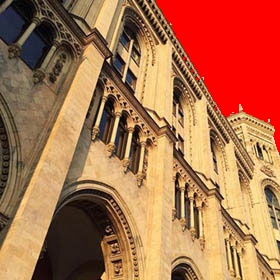## Segmentation

``````import "github.com/anthonynsimon/bild/segment"
``````

### Threshold

``````result := segment.Threshold(img, 128)
``````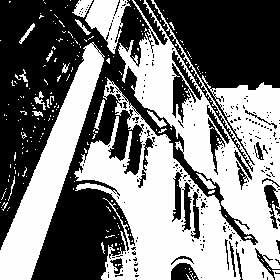## Transform

``````import "github.com/anthonynsimon/bild/transform"
``````

### Crop

``````// Source image is 280x280
result := transform.Crop(img, image.Rect(70,70,210,210))
``````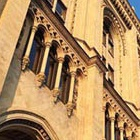### FlipH

``````result := transform.FlipH(img)
``````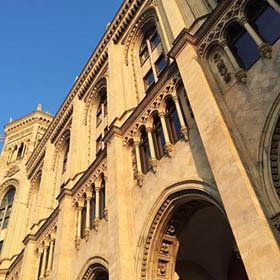### FlipV

``````result := transform.FlipV(img)
``````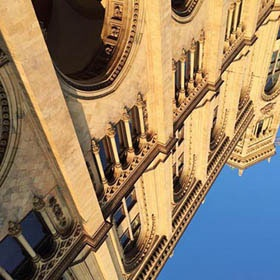### Resize Resampling Filters

``````result := transform.Resize(img, 280, 280, transform.Linear)
``````

### Rotate

``````// Options set to nil will use defaults (ResizeBounds set to false, Pivot at center)
result := transform.Rotate(img, -45.0, nil)
``````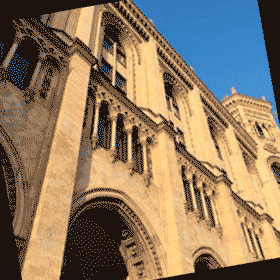``````// If ResizeBounds is set to true, the full rotation bounding area is used
result := transform.Rotate(img, -45.0, &transform.RotationOptions{ResizeBounds: true})
``````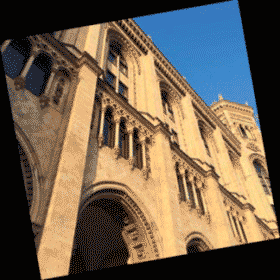``````// Pivot coordinates are set from the top-left corner
// Notice ResizeBounds being set to default (false)
result := transform.Rotate(img, -45.0, &transform.RotationOptions{Pivot: &image.Point{0, 0}})
``````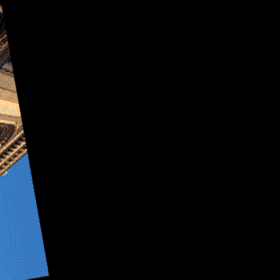### Shear Horizontal

``````result := transform.ShearH(img, 30)
``````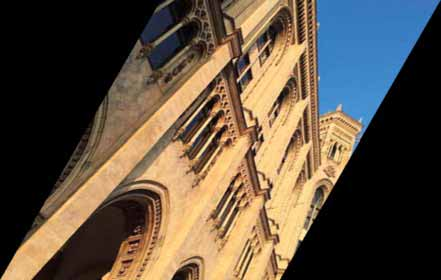### Shear Vertical

``````result := transform.ShearV(img, 30)
``````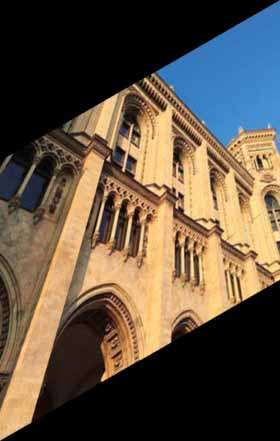### Translate

``````result := transform.Translate(img, 80, 0)
``````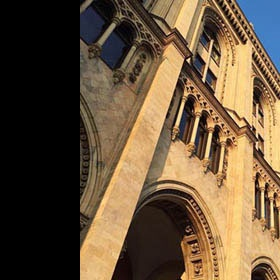## Contribute

Want to hack on the project? Any kind of contribution is welcome!

• Fork the project.
• Create a new branch.
• Make your changes and write tests when practical.
• Commit your changes to the new branch.
• Send a pull request, it will be reviewed shortly.

In case you want to add a feature, please create a new issue and briefly explain what the feature would consist of. For bugs or requests, before creating an issue please check if one has already been created for it.

## Changelog

Please see the changelog for more details.

Get A Weekly Email With Trending Projects For These Topics
No Spam. Unsubscribe easily at any time.
go (13,599
image-processing (463
algorithm (448
concurrency (182
effects (84
signal-processing (64
resize (42
parallelism (37
histogram (22